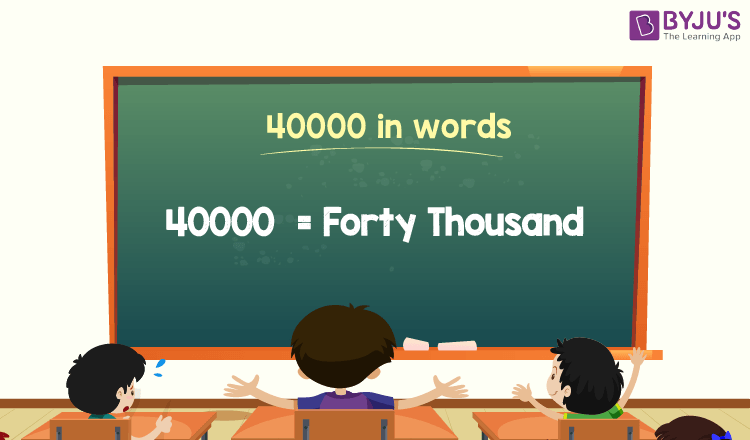# 40000 in Words

40000 in words is Forty Thousand. The number name of 40000 can be written using the ones, tens, hundreds, thousands place of a number. Thus, the place value chart helps to write the number 40000 in words.

 40000 in Words: Forty Thousand

This article explains how to write the number 40000 in words, and provides solved examples.

## How to Write 40000 in Words?The place value of a number can be used to write the number 40000 in words. Determine the place value of each digit in the given number using the place value chart. In this case, the number is 40000. So, identify the place value of each digit of 40000 to write the number’s name.

For a number 40000,

0 is the digit in one’s position

0 is the digit in ten’s position

0 is the digit in the hundred’s position

40 is the digit in the thousand’s position

Thus, 40000 is represented as follows on a place value chart:

 Thousands Hundreds Tens Ones 40 0 0 0

Therefore, the number 40000 in words is Forty Thousand.

### Examples

Example 1:

Find the value of 35000 + 5000. Describe the value in words.

Solution:

Given expression: 35000 + 5000

⇒ 35000 + 5000 = 40000

So, the value of 35000 + 5000 is 40000.

Hence, 40000 in words is forty thousand.

Example 2:

Determine the value of forty thousand plus two thousand in words.

Solution:

Forty thousand = 40000

Two thousand = 2000

Forty thousand plus two thousand = 40000 + 2000= 42000.

Hence, the value of forty thousand plus two thousand is forty-two thousand in words.

## Frequently Asked Questions on 40000 in Words

Q1

### Write 40000 in words.

40000 in words is forty thousand.

Q2

### Express the value 37000 + 3000 in words.

Simplifying 37000 + 3000, we get 40000. Hence, 40000 in words is forty thousand.

Q3

### How to write forty thousand in numbers?

Forty thousand in numbers is 40000.